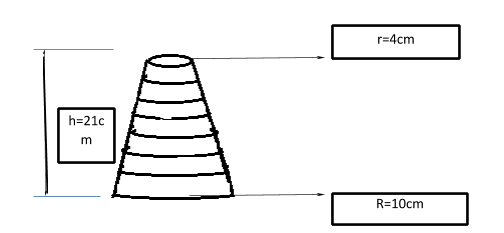QuestionAnswers

# In an experiment of mathematics frustum of cones is made up of seven rings, the radius of the uppermost ring is 4cm and the radius increases by 1cm so that radius of the last ring is 10cm, if the width of each ring is 3cm, find the volume of frustum.

Hint: A frustum is a part of a cone that is formed after removing the upper portion of the cone by a plane parallel to its base.Volume of a frustum= $\dfrac{1}{3}\pi h\left( {{r^2} + rR + {R^2}} \right)$

Volume of a frustum= $\dfrac{1}{3}\pi h\left( {{r^2} + rR + {R^2}} \right)$
$= \dfrac{1}{3} \times \dfrac{{22}}{7} \times 21\left( {{4^2} + 4 \times 10 + {{10}^2}} \right) \\ = 22\left( {16 + 40 + 100} \right) \\ = 22 \times 156 \\ = 3432c{m^3} \\$
Thus, volume of frustum is $3432 c{m^3}$.